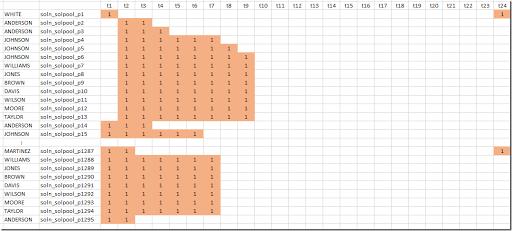## Tuesday, January 24, 2017

### Employee Scheduling III: generating all shifts using the Cplex solution pool

In (1) and (2) all possible shifts for a small Employee Scheduling problem are calculated by programming. In (3) we generate new shifts one by one using MIP model as part of a Column Generation algorithm. Here I want to show a different approach to generate all possible feasible shifts with a MIP model using the Cplex solution pool. So this is a post about “modeling instead of programming”.

The model is very similar to the sub-problem in the Column Generation scheme:

 binary variables   w(t) 'work at time t'   start(t) 'start of shift'   emp(e) 'select shift from employee e';positive variables   shiftlen  'length of shift';variable dummy;equations   startShift(t)    'start of shift: w(t) goes from 0 to 1'   oneShiftStart    'exactly one start of shift'   calcLen          'calculate length of shift'   oneEmployee      'pick one employee'   minLength        'observe minimum shift length'   maxLength        'observe maximum shift length'   feasibleShift(t) 'make sure shift is feasible'   dummyObj;startShift(t)..  start(t) =g= w(t) - w(t--1);oneShiftStart..  sum(t,start(t)) =e= 1;calcLen..        shiftLen =e= sum(t, w(t));oneEmployee..    sum(e, emp(e)) =e= 1;minLength..      shiftLen =g= sum(e, minlen(e)*emp(e));maxLength..      shiftLen =l= sum(e, maxlen(e)*emp(e));feasibleShift(t).. w(t) =l= sum(canwork(e,t),emp(e));dummyObj..       dummy =e= 0;option mip=cplex;$onecho > cplex.optSolnPoolAGap = 0.0SolnPoolIntensity = 4PopulateLim = 10000SolnPoolPop = 2solnpoolmerge solutions.gdx$offechomodel solpool /all/;solpool.optfile=1;solve solpool using mip minimizing dummy;

Actually the model is quite simpler as in the CG sub-problem we needed a rather complicated objective. Here we use a dummy objective as we just need to find a feasible shift.

This model finds a shift encoded by the binary variable $$w_t$$ and a corresponding employee denoted by $$emp_e \in \{0,1\}$$ (the selected employee will have a value of one).

The start of a shift is easily found: we need to look for a pattern $$w_t,w_{t+1} = 0, 1$$. Note that $$w_1$$ for $$t=1$$ is special: it may be a continuation of a shift with $$w_{24}=1$$. This is the reason why in the GAMS model we use “start(t) =g= w(t) - w(t--1)” with a circular lag operator --. To make sure shifts are contiguous we only need to require that $$\sum_t start_t \le 1$$ (note that $$\sum_t start_t = 0$$ can not happen, so we can also write $$\sum_t start_t = 1$$).

We check if a shift is only using allowed time slots in equation feasibleShift. Here we use the set canwork(e,t) indicating which time periods are allowed or forbidden for a given employee (see (2)). We require here that if canwork=0 then the corresponding $$w_t=0$$.

The Cplex solution pool (4) allows us to find all feasible integer solutions for a MIP very fast. This technology is used here to find all feasible shifts and indeed we find:We see we find the same 1295 shifts, although in a different order (the order is not important for our purposes).

Now we have found this complete collection of feasible shifts, we can solve our scheduling model:

 \begin{align}\min\>&\sum_s c_s x_s\\ &\sum_{s|E(e,s)} x_s \le 1 \>\forall e\\ &\sum_{s|S(s,t)} x_s \ge R_t \> \forall t\\ &x_s \in\{0,1\}\end{align}

With this approach we just use one MIP solve to find all feasible shifts and a second MIP solve to find the optimal schedule.

#### References

1. Loren Shure, Generating an Optimal Employee Work Schedule Using Integer Linear Programming, January 6, 2016, http://blogs.mathworks.com/loren/2016/01/06/generating-an-optimal-employee-work-schedule-using-integer-linear-programming/ describes the problem with a Matlab implementation.
2. Employee Scheduling I : Matlab vs GAMS, http://yetanothermathprogrammingconsultant.blogspot.com/2017/01/employee-scheduling-i-matlab-vs-gams.html has a GAMS version of the model in (1).
3. Employee Scheduling II : Column Generation, http://yetanothermathprogrammingconsultant.blogspot.com/2017/01/employee-scheduling-ii-column-generation.html
4. IBM, What is the solution pool?, http://www.ibm.com/support/knowledgecenter/SSSA5P_12.7.0/ilog.odms.cplex.help/CPLEX/UsrMan/topics/discr_optim/soln_pool/02_soln_pool_defn.html
5. Employee Scheduling IV: direct optimization, http://yetanothermathprogrammingconsultant.blogspot.com/2017/03/employee-scheduling-iv-direct.html